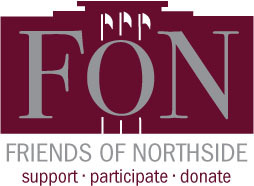# Friends of Northside

Tuesday, September 24
7:00 pm
Faculty Lounge

Friends of Northside (FON) is the “one-stop-shop” for NCP families to get involved. FON facilitates communication between parents and the school, organizes parent volunteers, plans and sponsors social/educational events, and raises much-needed funds to bridge the gap due to CPS budget cuts. FON supports the school’s mission to maintain and enhance the learning experience for all students of Northside College Prep High School.

To find out more about Friends of Northside, please visit their website HERE.

 table div table+table+table div table{width:100%;padding:0}table div table+table+table div table img{width:96.23%;padding:0;float:none}table div table+table+table div table td{width:100%;padding:0 1.88% 18px}/* styles */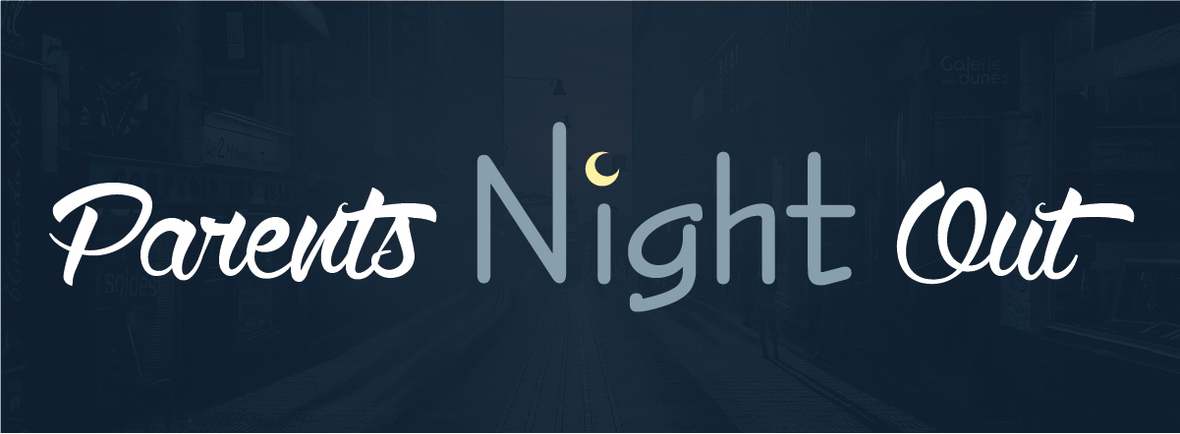# Fall Parents' Night Out

Thursday, September 26
6:00 pm
Via Veneto
6340 N. Lincoln Ave.

Join us for a Parents' Night Out at Via Veneto. Catch up with old friends and make new ones! There will be complimentary appetizers and a cash bar. Please sign up HERE so we can let the restaurant know how many people to expect.

 table div table+table+table+table+table+table div table{width:100%;padding:0}table div table+table+table+table+table+table div table img{width:96.23%;padding:0;float:none}table div table+table+table+table+table+table div table td{width:100%;padding:0 1.88% 18px}/* styles */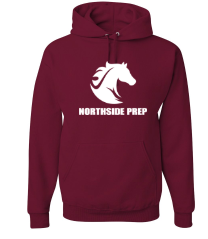# Northside Mustangs Spirit Wear Store

The Athletic Boosters have coordinated with All Seasons Screen Printing & Embroidery again this year to make NCP Spirit wear available to everyone online. The new mustang logo has now been incorporated into the offerings from last year. Shop for the latest gear HERE.

The store will be open until 11:59 pm on Sunday, September 29. Get your spirit wear today!

 table div table+table+table+table+table+table+table+table div table{width:100%;padding:0}table div table+table+table+table+table+table+table+table div table img{width:96.23%;padding:0;float:none}table div table+table+table+table+table+table+table+table div table td{width:100%;padding:0 1.88% 18px}/* styles */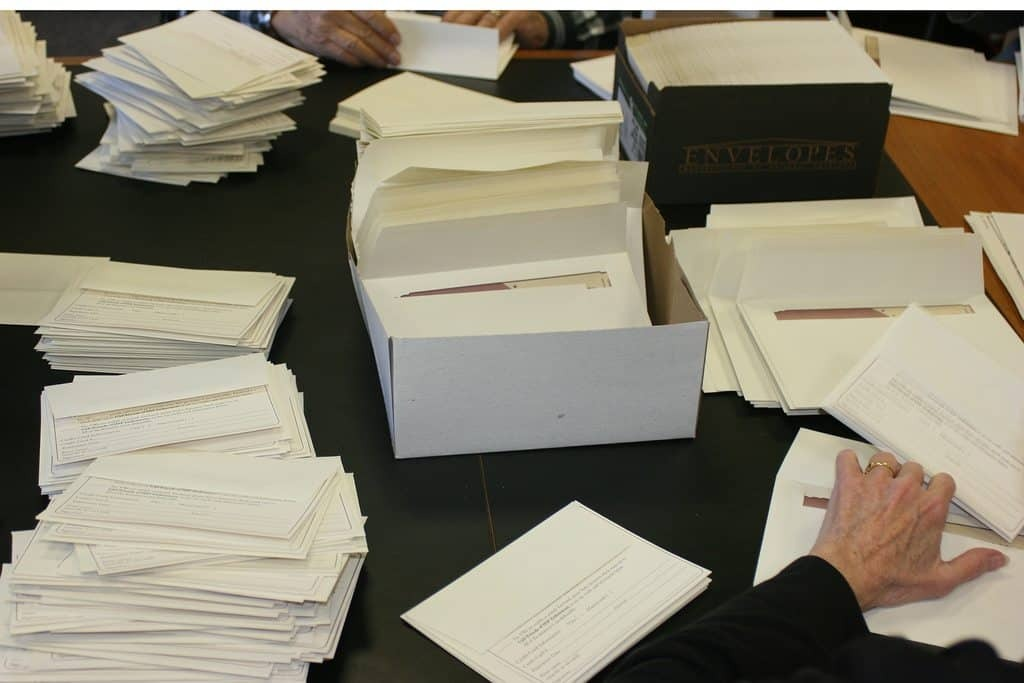# Annual Appeal Mailing

Wednesday, October 2
4:00 - 5:30 pm
(Please note the corrected start time.)

We're kicking off our Annual Appeal and need 10 volunteers to label and stuff envelopes for the mailing that goes to all NCP families and friends. No skills are required and it's guaranteed to be a good time! To sign up, click HERE.

 table div table+table+table+table+table+table+table+table+table+table div table{width:100%;padding:0}table div table+table+table+table+table+table+table+table+table+table div table img{width:96.23%;padding:0;float:none}table div table+table+table+table+table+table+table+table+table+table div table td{width:100%;padding:0 1.88% 18px}/* styles */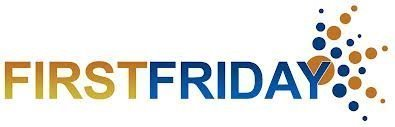# First Friday

Friday, October 4
8:00 - 10:00 am
Panera Bread, 6059 N. Lincoln Ave.

Friends of Northside gathers on the First Friday morning of every month for coffee and conversation. This casual get-together gives you a chance to meet and network with other parents. Feel free to come and go as it suits your schedule.

 table div table+table+table+table+table+table+table+table+table+table+table+table div table{width:100%;padding:0}table div table+table+table+table+table+table+table+table+table+table+table+table div table img{width:96.23%;padding:0;float:none}table div table+table+table+table+table+table+table+table+table+table+table+table div table td{width:100%;padding:0 1.88% 18px}/* styles */# Host Families Wanted

Wednesday, October 9-Friday, October 18

Northside College Prep will be hosting 12 students from Prague from October 9-18. We are looking for NCP families, preferably with a sophomore, junior, or senior, willing to host a student during that time and show them some of the amazing things that Northside and Chicago have to offer. If you are interested in hosting, please fill out an application form HERE. Information about the trip can be found in this Prague FAQ document. For additional information, please contact assistant principal Barry Smith.

 table div table+table+table+table+table+table+table+table+table+table+table+table+table+table div table{width:100%;padding:0}table div table+table+table+table+table+table+table+table+table+table+table+table+table+table div table img{width:96.23%;padding:0;float:none}table div table+table+table+table+table+table+table+table+table+table+table+table+table+table div table td{width:100%;padding:0 1.88% 18px}/* styles */# No School - CPS Day Off

Monday, October 14
Indigenous People's Day/Columbus Day

 table div table+table+table+table+table+table+table+table+table+table+table+table+table+table+table+table div table{width:100%;padding:0}table div table+table+table+table+table+table+table+table+table+table+table+table+table+table+table+table div table img{width:96.23%;padding:0;float:none}table div table+table+table+table+table+table+table+table+table+table+table+table+table+table+table+table div table td{width:100%;padding:0 1.88% 18px}/* styles */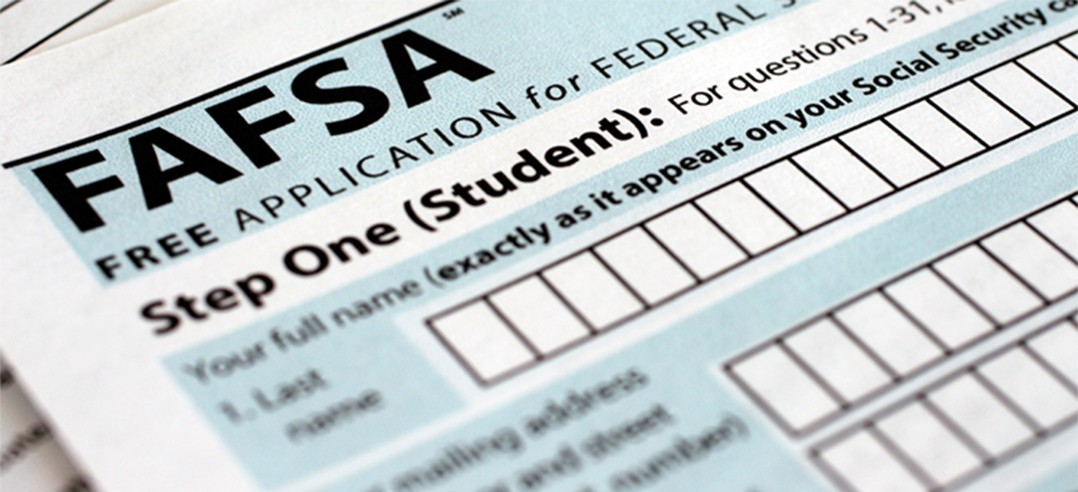# Financial Aid Completion Workshops

Tuesday, October 15
6:00 - 8:00 pm

The counseling department will be offering FAFSA Completion Workshops for English and Spanish-speaking parents and students on Tuesday, October 15 from 6:00 - 8:00 pm.

Additional student workshops will be held during the day for students who would like to start their FAFSA as soon as the application becomes available or if they would like one-on-one support.

Students and parents, please register HERE for the FAFSA Workshops. Also, students will be required to register for the workshops offered during the school day in their Enriching Student Portal as well.

## Presentations

ISAC Student FAFSA Workshop
Wednesday, October 2
Two sessions: 1:25 - 2:10 pm and 2:15 - 3:00 pm
Room 224

ISAC Parent and Student FAFSA Workshop (English & Spanish)
Tuesday, October 16
6:00 - 8:00 pm
Room 219

ISAC Student Financial Aid Follow-up
Wednesday, November 20
Two sessions: 1:25 - 2:10 pm and 2:15 - 3:00 pm
Room 224

 table div table+table+table+table+table+table+table+table+table+table+table+table+table+table+table+table+table+table div table{width:100%;padding:0}table div table+table+table+table+table+table+table+table+table+table+table+table+table+table+table+table+table+table div table img{width:96.23%;padding:0;float:none}table div table+table+table+table+table+table+table+table+table+table+table+table+table+table+table+table+table+table div table td{width:100%;padding:0 1.88% 18px}/* styles */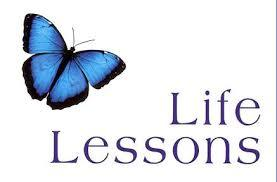# Guest Speakers Needed for Life Lessons Colloquium

Mr. Madia is looking for parents who would like to be a guest speaker for his life lessons colloquium.

He's looking for people in the field of finance, cooking, fashion, travel, hiring manager, auto, housing, life skills, etc. Please contact Mr. Madia directly to help.

 table div table+table+table+table+table+table+table+table+table+table+table+table+table+table+table+table+table+table+table+table div table{width:100%;padding:0}table div table+table+table+table+table+table+table+table+table+table+table+table+table+table+table+table+table+table+table+table div table img{width:96.23%;padding:0;float:none}table div table+table+table+table+table+table+table+table+table+table+table+table+table+table+table+table+table+table+table+table div table td{width:100%;padding:0 1.88% 18px}/* styles */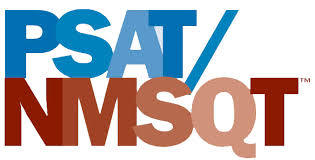# PSAT/NMST Exam

Wednesday, October 16
8:00 am

Northside College Prep sophomores and juniors will participate in the PSAT/NMSQT exam. On the day of the exam, students are expected to report to school no later than 8:00 am for a full day of school. More details about the exam will be shared with students and parents in the near future.

 table div table+table+table+table+table+table+table+table+table+table+table+table+table+table+table+table+table+table+table+table+table+table div table{width:100%;padding:0}table div table+table+table+table+table+table+table+table+table+table+table+table+table+table+table+table+table+table+table+table+table+table div table img{width:96.23%;padding:0;float:none}table div table+table+table+table+table+table+table+table+table+table+table+table+table+table+table+table+table+table+table+table+table+table div table td{width:100%;padding:0 1.88% 18px}/* styles */# FON Parent College Night

Thursday, October 17
6:30 - 8:00 pm
Second Floor Atrium

The Friends of Northside sponsored Parent College Night will be held Thursday, October 17 from 6:30 - 8:00 pm in the Second Floor Atrium. Come hear parents of recently graduated NCP students share their experiences and advice about the college search and application process. Please RSVP for the event HERE so we know how many people to expect.

 table div table+table+table+table+table+table+table+table+table+table+table+table+table+table+table+table+table+table+table+table+table+table+table+table div table{width:100%;padding:0}table div table+table+table+table+table+table+table+table+table+table+table+table+table+table+table+table+table+table+table+table+table+table+table+table div table img{width:96.23%;padding:0;float:none}table div table+table+table+table+table+table+table+table+table+table+table+table+table+table+table+table+table+table+table+table+table+table+table+table div table td{width:100%;padding:0 1.88% 18px}/* styles */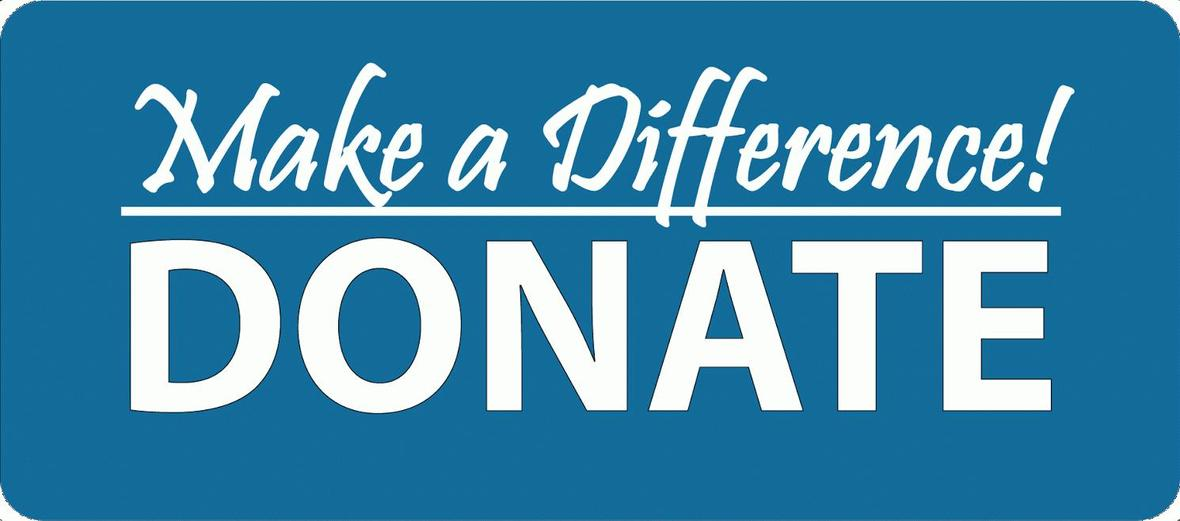# Donations Needed for Student Resource Closet

Friends of Northside has a resource closet available to NCP students. Currently, we are in need of:

▪ Individual snacks such as granola bars, nuts, and dried fruit
▪ Toothpaste and dental floss
▪ Hair conditioner
▪ Men’s and women’s underwear and socks
▪ Loose leaf and graph paper
▪ Spiral notebooks and binders
▪ Colored pencils
▪ Sanitizing wipes (Clorox type)
▪ Dryer sheets
▪ Laundry soap
 ▪ Individual snacks such as granola bars, nuts, and dried fruit
 ▪ Toothpaste and dental floss
 ▪ Hair conditioner
 ▪ Men’s and women’s underwear and socks
 ▪ Loose leaf and graph paper
 ▪ Spiral notebooks and binders
 ▪ Colored pencils
 ▪ Sanitizing wipes (Clorox type)
 ▪ Dryer sheets
 ▪ Laundry soap

All items must be new and unopened. No used clothing will be accepted. Please bring all items to the Counseling Office and set outside of Ms. Matthews' office.

Contact Nancy Liljedahl with any questions.

 table div table+table+table+table+table+table+table+table+table+table+table+table+table+table+table+table+table+table+table+table+table+table+table+table+table+table+table div table{width:100%;padding:0}table div table+table+table+table+table+table+table+table+table+table+table+table+table+table+table+table+table+table+table+table+table+table+table+table+table+table+table div table img{width:96.23%;padding:0;float:none}table div table+table+table+table+table+table+table+table+table+table+table+table+table+table+table+table+table+table+table+table+table+table+table+table+table+table+table div table td{width:100%;padding:0 1.88% 18px}/* styles */table div table+table+table+table+table+table+table+table+table+table+table+table+table+table+table+table+table+table+table+table+table+table+table+table+table+table+table+table div table{width:100%;padding:0}table div table+table+table+table+table+table+table+table+table+table+table+table+table+table+table+table+table+table+table+table+table+table+table+table+table+table+table+table div table img{width:96.23%;padding:0;float:none}table div table+table+table+table+table+table+table+table+table+table+table+table+table+table+table+table+table+table+table+table+table+table+table+table+table+table+table+table div table td{width:100%;padding:0 1.88% 18px}/* styles */# Important October Dates

▪ Wednesday, October 2 - ISAC Student FAFSA Workshop & Annual Appeal Mailing
▪ Friday, October 4 - First Friday
▪ Monday, October 14 - Indigenous People's Day/Columbus Day (no school)
▪ Tuesday, October 15 - LSC Meeting & FAFSA Completion Workshop
▪ Wednesday, October 16 - PSAT/NMST Exam & ISAC Parent and Student FAFSA Workshop
▪ Thursday, October 17 - FON Parent College Night & Athletic Booster Club meeting
▪ Friday, October 18 - Latino Fest
▪ Tuesday, October 22 - FON Meeting
▪ Friday, October 25 - Halloween Dance (sponsored by the Latin Club)
▪ Sunday, October 27 - NCP Open House
▪ Tuesday, October 29 - Halloween Concert
 ▪ Wednesday, October 2 - ISAC Student FAFSA Workshop & Annual Appeal Mailing
 ▪ Friday, October 4 - First Friday
 ▪ Monday, October 14 - Indigenous People's Day/Columbus Day (no school)
 ▪ Tuesday, October 15 - LSC Meeting & FAFSA Completion Workshop
 ▪ Wednesday, October 16 - PSAT/NMST Exam & ISAC Parent and Student FAFSA Workshop
 ▪ Thursday, October 17 - FON Parent College Night & Athletic Booster Club meeting
 ▪ Friday, October 18 - Latino Fest
 ▪ Tuesday, October 22 - FON Meeting
 ▪ Friday, October 25 - Halloween Dance (sponsored by the Latin Club)
 ▪ Sunday, October 27 - NCP Open House
 ▪ Tuesday, October 29 - Halloween Concert
 table div table+table+table+table+table+table+table+table+table+table+table+table+table+table+table+table+table+table+table+table+table+table+table+table+table+table+table+table+table+table+table div table{width:100%;padding:0}table div table+table+table+table+table+table+table+table+table+table+table+table+table+table+table+table+table+table+table+table+table+table+table+table+table+table+table+table+table+table+table div table img{width:96.23%;padding:0;float:none}table div table+table+table+table+table+table+table+table+table+table+table+table+table+table+table+table+table+table+table+table+table+table+table+table+table+table+table+table+table+table+table div table td{width:100%;padding:0 1.88% 18px}/* styles */# This Week in Sports

Come out and support our student athletes!

## Women's Tennis

▪ Monday, September 23, 5:00 pm (V) vs. Taft at NCP
 ▪ Monday, September 23, 5:00 pm (V) vs. Taft at NCP

## Men's Soccer

▪ Monday, September 23, 4:30 pm (V) and 6:00 pm (JV) vs. Whitney Young at Winnemac Stadium
 ▪ Monday, September 23, 4:30 pm (V) and 6:00 pm (JV) vs. Whitney Young at Winnemac Stadium

## Women's Volleyball

▪ Tuesday, September 24, 5:00 pm (V) and 6:00 pm (JV) vs. Kennedy at NCP
▪ Wednesday, September 25, 5:00 pm (F-A), 5:00 pm (JV), 6:00 pm (F-B), and 6:00 pm (V) vs. De La Salle Institute at De La Salle Institute
▪ Thursday, September 26, 5:00 pm (F-A), 5:00 pm (V), 6:00 pm (F-B), and 6:00 pm (JV) vs. Lane Tech at Lane Tech High School Saturday, September 28, 8:00 am (V) vs. TBA at NCP
 ▪ Tuesday, September 24, 5:00 pm (V) and 6:00 pm (JV) vs. Kennedy at NCP
 ▪ Wednesday, September 25, 5:00 pm (F-A), 5:00 pm (JV), 6:00 pm (F-B), and 6:00 pm (V) vs. De La Salle Institute at De La Salle Institute
 ▪ Thursday, September 26, 5:00 pm (F-A), 5:00 pm (V), 6:00 pm (F-B), and 6:00 pm (JV) vs. Lane Tech at Lane Tech High School Saturday, September 28, 8:00 am (V) vs. TBA at NCP

## Women's Softball

▪ Monday, September 23, 4:30 pm (V) vs. Taft at TBA
 ▪ Monday, September 23, 4:30 pm (V) vs. Taft at TBA

## Women's Swimming

▪ Thursday, September 26, 5:00 pm (V) vs. Whitney Young at Whitney Young
 ▪ Thursday, September 26, 5:00 pm (V) vs. Whitney Young at Whitney Young

## Women's Golf

▪ Wednesday, September 25, 8:00 am (V) vs. Chicago Public at Billy Caldwell
▪ Sunday, September 29, 10:00 am (V) vs. Chicago Public at Indian Boundary
 ▪ Wednesday, September 25, 8:00 am (V) vs. Chicago Public at Billy Caldwell
 ▪ Sunday, September 29, 10:00 am (V) vs. Chicago Public at Indian Boundary

## Men's Golf

▪ Tuesday, September 24, 3:00 pm (V) vs. Chicago Public at Billy Caldwell
▪ Thursday, September 26, 10:00 am (V) vs. Chicago Public at Indian Boundary
 ▪ Tuesday, September 24, 3:00 pm (V) vs. Chicago Public at Billy Caldwell
 ▪ Thursday, September 26, 10:00 am (V) vs. Chicago Public at Indian Boundary

## Women's Cross Country

▪ Tuesday, September 24, 4:30 pm (V) vs. Mather at NCP
▪ Saturday, September 28, 8:30 am (V) vs. Latin and Loyola at Montrose Beach
 ▪ Tuesday, September 24, 4:30 pm (V) vs. Mather at NCP
 ▪ Saturday, September 28, 8:30 am (V) vs. Latin and Loyola at Montrose Beach

## Men's Cross Country

▪ Tuesday, September 24, 4:30 pm (V) vs. Mather at NCP
▪ Saturday, September 28, 8:30 am (V) vs. Latin and Loyola at Montrose Beach
 ▪ Tuesday, September 24, 4:30 pm (V) vs. Mather at NCP
 ▪ Saturday, September 28, 8:30 am (V) vs. Latin and Loyola at Montrose Beach
 /* styles */ Visit the NCP Athletics website for additional information about Northside teams.
 table div table+table+table+table+table+table+table+table+table+table+table+table+table+table+table+table+table+table+table+table+table+table+table+table+table+table+table+table+table+table+table+table+table+table div table{width:100%;padding:0}table div table+table+table+table+table+table+table+table+table+table+table+table+table+table+table+table+table+table+table+table+table+table+table+table+table+table+table+table+table+table+table+table+table+table div table img{width:96.23%;padding:0;float:none}table div table+table+table+table+table+table+table+table+table+table+table+table+table+table+table+table+table+table+table+table+table+table+table+table+table+table+table+table+table+table+table+table+table+table div table td{width:100%;padding:0 1.88% 18px}/* styles */# No Experience Necessary

Make a commitment to Northside by offering your time for any of these rewarding on-going volunteer positions. Email Friends of Northside to indicate your interest or ask questions.

## Graphic Designer

Provide professional guidance and materials for various design needs throughout the year.

## Volunteer Coordinator

Manage and update our online volunteer system as needed throughout the year.

## Spring Benefit Committee

The Spring Benefit is NCP’s major spring fundraiser. Get involved, get to know NCP staff and parents, and make a profound impact on Northside College Prep.

When is the Spring Benefit?
Friday, March 13, 2020

What’s the time commitment for the team?

What roles are available?
* Entertainment Chair--one or two people to determine and arrange the entertainment for the Spring Benefit.
* Auction Item Solicitors--at least five people to solicit auction items.
* Printed Materials Chair--one or two people to design and produce all the printed materials.

Email Debra Swan, Event Chair, for more information or to join the team.

 table div table+table+table+table+table+table+table+table+table+table+table+table+table+table+table+table+table+table+table+table+table+table+table+table+table+table+table+table+table+table+table+table+table+table+table+table+table+table div table{width:100%;padding:0}table div table+table+table+table+table+table+table+table+table+table+table+table+table+table+table+table+table+table+table+table+table+table+table+table+table+table+table+table+table+table+table+table+table+table+table+table+table+table div table img{width:96.23%;padding:0;float:none}table div table+table+table+table+table+table+table+table+table+table+table+table+table+table+table+table+table+table+table+table+table+table+table+table+table+table+table+table+table+table+table+table+table+table+table+table+table+table div table td{width:100%;padding:0 1.88% 18px}/* styles */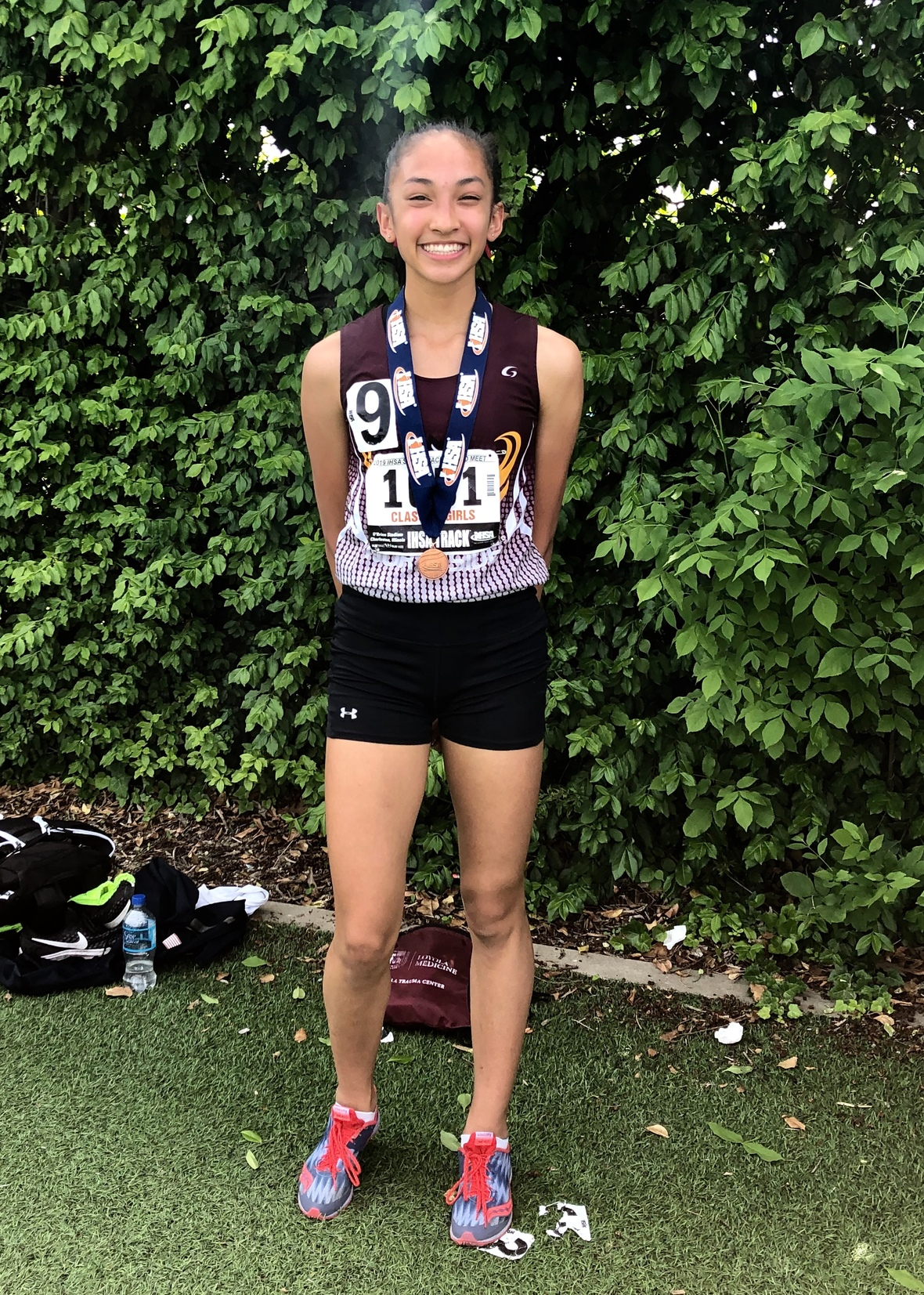# NCP Cross-Country Runners Shine at First to the Finish Meet

On Saturday, September 14, our men's and women's cross country teams competed in the First to the Finish Invitational meet at Dettweiler Park in Peoria, IL. This is the location for the IHSA State Finals and is considered a high profile event, showcasing some of the best runners in the state.

Northside sophomore Elia Ton-That was mentioned in a runnerspace.com article on September 12 as a “runner to watch”. She did not disappoint - Elia finished in 7th place in the Class 2A race, which featured 417 runners!

On the men's side, junior Ezra Hill continued his streak of strong finishes placing 46th out of 506 runners!

# Do you have news or team results to share with the Northside parent community?

 table div table+table+table+table+table+table+table+table+table+table+table+table+table+table+table+table+table+table+table+table+table+table+table+table+table+table+table+table+table+table+table+table+table+table+table+table+table+table+table+table+table+table div table{width:100%;padding:0}table div table+table+table+table+table+table+table+table+table+table+table+table+table+table+table+table+table+table+table+table+table+table+table+table+table+table+table+table+table+table+table+table+table+table+table+table+table+table+table+table+table+table div table img{width:96.23%;padding:0;float:none}table div table+table+table+table+table+table+table+table+table+table+table+table+table+table+table+table+table+table+table+table+table+table+table+table+table+table+table+table+table+table+table+table+table+table+table+table+table+table+table+table+table+table div table td{width:100%;padding:0 1.88% 18px}/* styles */# September and Early October College Visits at Northside

Monday, September 23
Massachusetts Institute of Technology, 3:15 pm

Tuesday, September 24
Nova Southeastern University, 10:38 am
St. John's University, 10:38 am
Saint Louis University, 12:26 pm

Wednesday, September 25
Calvin University, 10:40 am
Suffolk University, 11:20 am
*Kalamazoo College, 1:25 pm (register via Enriching Students)
Indiana University at Bloomington, 3:15 pm

Thursday, September 26
DePauw University, 9:48 am
Missouri State University, 12:26 pm
American University, 3:15 pm

Friday, September 27
St. Olaf College, 9:48 am

Monday, September 30
Barnard College, 9:48 am
University of California, Berkeley, 9:48 am
Middlebury College, 9:48 am
Wesleyan University, 10:38 am
Lehigh University, 11:36 am
Temple University, 3:15 pm

Tuesday, October 1
Davidson College, 9:48 am
Elon University, 9:48 am
Taylor University, 9:48 am
Amherst College, 11:36 am
Dominican University, 12:26 PM
Pennsylvania State University, 12:26 pm
Williams College, 12:26 pm
University of Chicago, 3:15 pm

Wednesday, October 2
Providence College,10:40 am
Santa Clara University,11:20 am
Willamette University,11:20 am
University of Tulsa, 12:40 pm
Niagara University, 3:15 pm

Thursday, October 3
Drake University, 9:48 am
Mercer University, 9:48 AM
University of Exeter, 10:38 AM
California Lutheran University, 12:26 pm
Ecole Hoteliere de Lausanne,12:26 pm
Hope College, 12:26 pm
Wentworth Institute of Technology, 12:26 pm
Northeastern University, 3:15 pm

Friday, October 4
Rose-Hulman Institute of Technology, 9:48 am
Stevens Institute of Technology, 9:48 am
The University of Alabama, 10:15 am
University of Dayton, 10:15 am
Indiana State University, 10:15 am
Indiana University - Purdue University Indianapolis, 10:15 am
The University of Oklahoma, 10:15 am
University of New Orleans, 10:38 am
Regent's University London, 11:36 am
Bowdoin College, 12:26 pm
Embry-Riddle Aeronautical University - Daytona Beach, 12:26 pm
Grinnell College, 3:15 pm

The list of college visits is updated frequently. For the most current information, parents of juniors and seniors should log into their parent account for Naviance.

Students are highly encouraged to meet with the reps who take the time to visit Northside. Oftentimes these are the same regional reps who will be reading your child's application! Students may ask a teacher to be briefly excused from class to meet a college representative.

 table div table+table+table+table+table+table+table+table+table+table+table+table+table+table+table+table+table+table+table+table+table+table+table+table+table+table+table+table+table+table+table+table+table+table+table+table+table+table+table+table+table+table+table+table+table div table{width:100%;padding:0}table div table+table+table+table+table+table+table+table+table+table+table+table+table+table+table+table+table+table+table+table+table+table+table+table+table+table+table+table+table+table+table+table+table+table+table+table+table+table+table+table+table+table+table+table+table div table img{width:96.23%;padding:0;float:none}table div table+table+table+table+table+table+table+table+table+table+table+table+table+table+table+table+table+table+table+table+table+table+table+table+table+table+table+table+table+table+table+table+table+table+table+table+table+table+table+table+table+table+table+table+table div table td{width:100%;padding:0 1.88% 18px}/* styles */# CPS Scholarship Notifications and College Events Around the City

For more details about the upcoming events and to access the most recent updates, view the College Calendar on the Northside Prep Counseling Website HERE.

 table div table+table+table+table+table+table+table+table+table+table+table+table+table+table+table+table+table+table+table+table+table+table+table+table+table+table+table+table+table+table+table+table+table+table+table+table+table+table+table+table+table+table+table+table+table+table+table div table{width:100%;padding:0}table div table+table+table+table+table+table+table+table+table+table+table+table+table+table+table+table+table+table+table+table+table+table+table+table+table+table+table+table+table+table+table+table+table+table+table+table+table+table+table+table+table+table+table+table+table+table+table div table img{width:96.23%;padding:0;float:none}table div table+table+table+table+table+table+table+table+table+table+table+table+table+table+table+table+table+table+table+table+table+table+table+table+table+table+table+table+table+table+table+table+table+table+table+table+table+table+table+table+table+table+table+table+table+table+table div table td{width:100%;padding:0 1.88% 18px}/* styles */# National Association for College Admission Counseling (NACAC) College Fair

Saturday, October 5
11:00 am – 3:00 pm

McCormick Place- Lakeside Center
2301 S. King Dr.

Free and open to the public, National College Fairs allow students to interact with admission representatives from a wide range of post-secondary institutions.

 table div table+table+table+table+table+table+table+table+table+table+table+table+table+table+table+table+table+table+table+table+table+table+table+table+table+table+table+table+table+table+table+table+table+table+table+table+table+table+table+table+table+table+table+table+table+table+table+table+table div table{width:100%;padding:0}table div table+table+table+table+table+table+table+table+table+table+table+table+table+table+table+table+table+table+table+table+table+table+table+table+table+table+table+table+table+table+table+table+table+table+table+table+table+table+table+table+table+table+table+table+table+table+table+table+table div table img{width:96.23%;padding:0;float:none}table div table+table+table+table+table+table+table+table+table+table+table+table+table+table+table+table+table+table+table+table+table+table+table+table+table+table+table+table+table+table+table+table+table+table+table+table+table+table+table+table+table+table+table+table+table+table+table+table+table div table td{width:100%;padding:0 1.88% 18px}/* styles */# 100 Black Men of Chicago, Inc. - 17th Annual College Scholarship Fair

Saturday, October 5
9:00 am - 2:00 pm
University of Illinois, at Chicago - Pavilion
525 S. Racine Ave.

Since 2003, the 100BMC has hosted an annual College Scholarship Fair with up to 200 Colleges and Universities interacting with nearly 5000 students, parents and educational partners.

Students can register HERE.

 table div table+table+table+table+table+table+table+table+table+table+table+table+table+table+table+table+table+table+table+table+table+table+table+table+table+table+table+table+table+table+table+table+table+table+table+table+table+table+table+table+table+table+table+table+table+table+table+table+table+table+table div table{width:100%;padding:0}table div table+table+table+table+table+table+table+table+table+table+table+table+table+table+table+table+table+table+table+table+table+table+table+table+table+table+table+table+table+table+table+table+table+table+table+table+table+table+table+table+table+table+table+table+table+table+table+table+table+table+table div table img{width:96.23%;padding:0;float:none}table div table+table+table+table+table+table+table+table+table+table+table+table+table+table+table+table+table+table+table+table+table+table+table+table+table+table+table+table+table+table+table+table+table+table+table+table+table+table+table+table+table+table+table+table+table+table+table+table+table+table+table div table td{width:100%;padding:0 1.88% 18px}/* styles */# Tufts University Admissions: Who Gets In and Why

Sunday, October 6, 02:00 - 04:00 pm
Hilton Garden Inn Chicago North Shore/Evanston
1818 Maple Ave
Evanston, IL 60201

Please join Rachel Brown, Associate Director of Admissions, and Evelyn Ocampo, Admissions Counselor, for a look inside a selective college admissions process. Insight into how and why admissions decisions are made will be revealed during this interactive workshop. The event is open to students, families, and school counselors.

Students can register HERE for the workshop.

 table div table+table+table+table+table+table+table+table+table+table+table+table+table+table+table+table+table+table+table+table+table+table+table+table+table+table+table+table+table+table+table+table+table+table+table+table+table+table+table+table+table+table+table+table+table+table+table+table+table+table+table+table+table div table{width:100%;padding:0}table div table+table+table+table+table+table+table+table+table+table+table+table+table+table+table+table+table+table+table+table+table+table+table+table+table+table+table+table+table+table+table+table+table+table+table+table+table+table+table+table+table+table+table+table+table+table+table+table+table+table+table+table+table div table img{width:96.23%;padding:0;float:none}table div table+table+table+table+table+table+table+table+table+table+table+table+table+table+table+table+table+table+table+table+table+table+table+table+table+table+table+table+table+table+table+table+table+table+table+table+table+table+table+table+table+table+table+table+table+table+table+table+table+table+table+table+table div table td{width:100%;padding:0 1.88% 18px}/* styles */Thursday, September 26
App Closes: Quest Bridge National Match Program

Friday, September 27
App Closes: Chicago Aldermanic Black Caucus Scholarship

Monday, September 30
App Closes: Cirkled In - "No Sweat" Scholarship
5:30 pm - Registration opens: CPS Student/Parent Scholarship Information Session

Thursday, October 10
App Closes: City Treasurer's Hispanic Heritage Month Scholarship
7:00 pm - Boston University Reception in Winnetka

Tuesday, October 15
App Closes: Hispanic Heritage Foundation’s Youth Awards

 table div table+table+table+table+table+table+table+table+table+table+table+table+table+table+table+table+table+table+table+table+table+table+table+table+table+table+table+table+table+table+table+table+table+table+table+table+table+table+table+table+table+table+table+table+table+table+table+table+table+table+table+table+table+table+table div table{width:100%;padding:0}table div table+table+table+table+table+table+table+table+table+table+table+table+table+table+table+table+table+table+table+table+table+table+table+table+table+table+table+table+table+table+table+table+table+table+table+table+table+table+table+table+table+table+table+table+table+table+table+table+table+table+table+table+table+table+table div table img{width:96.23%;padding:0;float:none}table div table+table+table+table+table+table+table+table+table+table+table+table+table+table+table+table+table+table+table+table+table+table+table+table+table+table+table+table+table+table+table+table+table+table+table+table+table+table+table+table+table+table+table+table+table+table+table+table+table+table+table+table+table+table+table div table td{width:100%;padding:0 1.88% 18px}/* styles */# New to Parent Portal?

1. Obtain your student's PIN, which should be on your acceptance letter or school welcome letter.
2. Create an account HERE.
 1 Obtain your student's PIN, which should be on your acceptance letter or school welcome letter.
 2 Create an account HERE.

PINs can only be provided to the parent/guardian in person or by mail to the address on file. They cannot be provided by phone or email, or to a student. To request your PIN, please contact Sonia Fernandez in the Counseling Office.

 table div table+table+table+table+table+table+table+table+table+table+table+table+table+table+table+table+table+table+table+table+table+table+table+table+table+table+table+table+table+table+table+table+table+table+table+table+table+table+table+table+table+table+table+table+table+table+table+table+table+table+table+table+table+table+table+table+table div table{width:100%;padding:0}table div table+table+table+table+table+table+table+table+table+table+table+table+table+table+table+table+table+table+table+table+table+table+table+table+table+table+table+table+table+table+table+table+table+table+table+table+table+table+table+table+table+table+table+table+table+table+table+table+table+table+table+table+table+table+table+table+table div table img{width:96.23%;padding:0;float:none}table div table+table+table+table+table+table+table+table+table+table+table+table+table+table+table+table+table+table+table+table+table+table+table+table+table+table+table+table+table+table+table+table+table+table+table+table+table+table+table+table+table+table+table+table+table+table+table+table+table+table+table+table+table+table+table+table+table div table td{width:100%;padding:0 1.88% 18px}/* styles */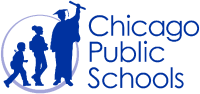# CPS Volunteer Requirements

According to CPS policy, anybody who is going to be in contact with students must be approved as a CPS volunteer. There are two levels of volunteers, Level 1 and Level 2.

## Level I Volunteers

(Please allow 8-12 weeks for the entire process)
A fingerprint criminal background check is required for all Level I Volunteers.

A Level I volunteer is:

1. A parent providing volunteer service in their child’s school or classroom for 10 or more hours/week;
2. An individual providing volunteer service for 5 or more hours/week at a school where s/he does not have a child enrolled;
3. Any individual chaperoning an overnight school-sponsored trip, regardless of the hours/week that the volunteer serves (See also the Student Travel Policy).
 1 A parent providing volunteer service in their child’s school or classroom for 10 or more hours/week;
 2 An individual providing volunteer service for 5 or more hours/week at a school where s/he does not have a child enrolled;
 3 Any individual chaperoning an overnight school-sponsored trip, regardless of the hours/week that the volunteer serves (See also the Student Travel Policy).

## Level II Volunteers

(This process can be completed in a day & is required even of volunteers for Friends of Northside events)
A fingerprint criminal background check is not required for Level II Volunteers.

A Level II Volunteer is:

1. A parent providing supervised volunteer service in their child’s school or classroom for less than 10 hours/week;
2. Any individual providing volunteer service for less than 5 hours/week at a school where s/he does not have a child enrolled;
3. An individual providing incidental volunteer service with no ongoing individualized interaction with a student(s) including those who speak at a class/assembly, judge academic competitions, give musical performance, participate in the “Principal for a Day” program, job shadowing event or other one-time event provided where there is direct supervision of the activity/event by regular school employees;
4. A parent accompanying his/her child’s class on a one-day field trip or another type of extracurricular activity that does not involve an overnight stay;
5. An individual providing volunteer service on projects/activities involving no or nominal contact with children (home-based volunteer activities, volunteers serving at Central or Network office).
 1 A parent providing supervised volunteer service in their child’s school or classroom for less than 10 hours/week;
 2 Any individual providing volunteer service for less than 5 hours/week at a school where s/he does not have a child enrolled;
 3 An individual providing incidental volunteer service with no ongoing individualized interaction with a student(s) including those who speak at a class/assembly, judge academic competitions, give musical performance, participate in the “Principal for a Day” program, job shadowing event or other one-time event provided where there is direct supervision of the activity/event by regular school employees;
 4 A parent accompanying his/her child’s class on a one-day field trip or another type of extracurricular activity that does not involve an overnight stay;
 5 An individual providing volunteer service on projects/activities involving no or nominal contact with children (home-based volunteer activities, volunteers serving at Central or Network office).

To become a volunteer, please visit CPS' Volunteer website and follow the instructions. There is also a link on Northside's website on the Parents page Northside Volunteers.

Assistant Principal, Kaitlin O'Hare is Northside's Volunteer Coordinator. After applying to become a volunteer, you must visit the school and show Ms. O'Hare your ID, so she can approve you to move forward in the volunteer process. For Level II volunteers, that is the only step you must take. For Level 1, you will receive further instructions from CPS.

 table div table+table+table+table+table+table+table+table+table+table+table+table+table+table+table+table+table+table+table+table+table+table+table+table+table+table+table+table+table+table+table+table+table+table+table+table+table+table+table+table+table+table+table+table+table+table+table+table+table+table+table+table+table+table+table+table+table+table+table div table{width:100%;padding:0}table div table+table+table+table+table+table+table+table+table+table+table+table+table+table+table+table+table+table+table+table+table+table+table+table+table+table+table+table+table+table+table+table+table+table+table+table+table+table+table+table+table+table+table+table+table+table+table+table+table+table+table+table+table+table+table+table+table+table+table div table img{width:96.23%;padding:0;float:none}table div table+table+table+table+table+table+table+table+table+table+table+table+table+table+table+table+table+table+table+table+table+table+table+table+table+table+table+table+table+table+table+table+table+table+table+table+table+table+table+table+table+table+table+table+table+table+table+table+table+table+table+table+table+table+table+table+table+table+table div table td{width:100%;padding:0 1.88% 18px}/* styles */# FON Connect

Friends of Northside uses a customer/constituent relationship management (CRM) system known as FON Connect that allows our parent volunteer organization to work from one database for events. It is not a CPS database and is completely different and separate from the CPS Parent Portal and Northside's EPay website.

FON Connect also serves as the NCP Family Directory and allows users to review their donation history, update their contact information, register for upcoming events, and more. Each parent has a separate account. Transactions, including donations, are recorded in the account of the parent who initiated the transaction or donation.

If you’re new to FON Connect, you'll need to set up a login and password. To do so:

 table div table+table+table+table+table+table+table+table+table+table+table+table+table+table+table+table+table+table+table+table+table+table+table+table+table+table+table+table+table+table+table+table+table+table+table+table+table+table+table+table+table+table+table+table+table+table+table+table+table+table+table+table+table+table+table+table+table+table+table+table+table div table{width:100%;padding:0}table div table+table+table+table+table+table+table+table+table+table+table+table+table+table+table+table+table+table+table+table+table+table+table+table+table+table+table+table+table+table+table+table+table+table+table+table+table+table+table+table+table+table+table+table+table+table+table+table+table+table+table+table+table+table+table+table+table+table+table+table+table div table img{width:96.23%;padding:0;float:none}table div table+table+table+table+table+table+table+table+table+table+table+table+table+table+table+table+table+table+table+table+table+table+table+table+table+table+table+table+table+table+table+table+table+table+table+table+table+table+table+table+table+table+table+table+table+table+table+table+table+table+table+table+table+table+table+table+table+table+table+table+table div table td{width:100%;padding:0 1.88% 18px}/* styles */# Parking Lot Safety

Our NCP school parking lot is a bustling place before and after school. Families dropping off and picking up students are asked to be mindful of both safety and courtesy. Please assist us by ensuring that traffic lanes stay clear during drop-off and pick-up times and by following the parking lot procedures.

# Parking Lot Procedures

ALL vehicles MUST exit onto Kedzie. You may enter the parking lot from Kedzie or Bryn Mawr, but regardless of where you enter, all cars must exit onto Kedzie. For the safety of our students and the rest of the Northside community, please do not exit onto Bryn Mawr.

The first few days will be busy at drop-off and pick-up. Please, for the safety of all involved, plan to give yourself enough time for drop-off and pick-up and please be patient while in the parking lot. Pulling over to the curb on the right as your student enters or exits your vehicle keeps students safe and traffic flow efficient. Our safety team will be actively working to ensure that students arrive and depart safely each day and that vehicles in school lots remain damage-free.

 table div table+table+table+table+table+table+table+table+table+table+table+table+table+table+table+table+table+table+table+table+table+table+table+table+table+table+table+table+table+table+table+table+table+table+table+table+table+table+table+table+table+table+table+table+table+table+table+table+table+table+table+table+table+table+table+table+table+table+table+table+table+table+table div table{width:100%;padding:0}table div table+table+table+table+table+table+table+table+table+table+table+table+table+table+table+table+table+table+table+table+table+table+table+table+table+table+table+table+table+table+table+table+table+table+table+table+table+table+table+table+table+table+table+table+table+table+table+table+table+table+table+table+table+table+table+table+table+table+table+table+table+table+table div table img{width:96.23%;padding:0;float:none}table div table+table+table+table+table+table+table+table+table+table+table+table+table+table+table+table+table+table+table+table+table+table+table+table+table+table+table+table+table+table+table+table+table+table+table+table+table+table+table+table+table+table+table+table+table+table+table+table+table+table+table+table+table+table+table+table+table+table+table+table+table+table+table div table td{width:100%;padding:0 1.88% 18px}/* styles */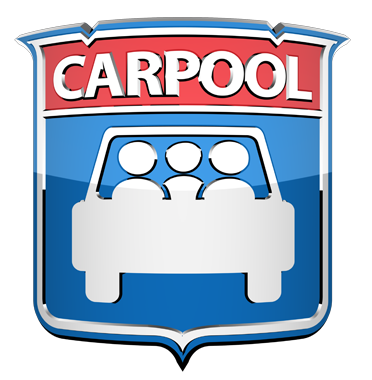# NCP Carpool Connection!

If you're interested in carpooling to school, add your address to the 2019-2020 NCP Carpool map and reach out to contact a potential carpool family on the map. Find the map HERE or on the Welcome page of FON Connect.

 table div table+table+table+table+table+table+table+table+table+table+table+table+table+table+table+table+table+table+table+table+table+table+table+table+table+table+table+table+table+table+table+table+table+table+table+table+table+table+table+table+table+table+table+table+table+table+table+table+table+table+table+table+table+table+table+table+table+table+table+table+table+table+table+table+table div table{width:100%;padding:0}table div table+table+table+table+table+table+table+table+table+table+table+table+table+table+table+table+table+table+table+table+table+table+table+table+table+table+table+table+table+table+table+table+table+table+table+table+table+table+table+table+table+table+table+table+table+table+table+table+table+table+table+table+table+table+table+table+table+table+table+table+table+table+table+table+table div table img{width:96.23%;padding:0;float:none}table div table+table+table+table+table+table+table+table+table+table+table+table+table+table+table+table+table+table+table+table+table+table+table+table+table+table+table+table+table+table+table+table+table+table+table+table+table+table+table+table+table+table+table+table+table+table+table+table+table+table+table+table+table+table+table+table+table+table+table+table+table+table+table+table+table div table td{width:100%;padding:0 1.88% 18px}/* styles */# Attention, Parents of CTA Commuters

Did you know that the #82 and #92 buses pick up students in front of Northside at the end of the school day? The Kimball Brown line station is also close to school at Kimball and Lawrence.

These are just a few of the ways students can use public transportation to commute to school. More information on routes for your student can be found at the CTA website HERE.

 table div table+table+table+table+table+table+table+table+table+table+table+table+table+table+table+table+table+table+table+table+table+table+table+table+table+table+table+table+table+table+table+table+table+table+table+table+table+table+table+table+table+table+table+table+table+table+table+table+table+table+table+table+table+table+table+table+table+table+table+table+table+table+table+table+table+table+table div table{width:100%;padding:0}table div table+table+table+table+table+table+table+table+table+table+table+table+table+table+table+table+table+table+table+table+table+table+table+table+table+table+table+table+table+table+table+table+table+table+table+table+table+table+table+table+table+table+table+table+table+table+table+table+table+table+table+table+table+table+table+table+table+table+table+table+table+table+table+table+table+table+table div table img{width:96.23%;padding:0;float:none}table div table+table+table+table+table+table+table+table+table+table+table+table+table+table+table+table+table+table+table+table+table+table+table+table+table+table+table+table+table+table+table+table+table+table+table+table+table+table+table+table+table+table+table+table+table+table+table+table+table+table+table+table+table+table+table+table+table+table+table+table+table+table+table+table+table+table+table div table td{width:100%;padding:0 1.88% 18px}/* styles */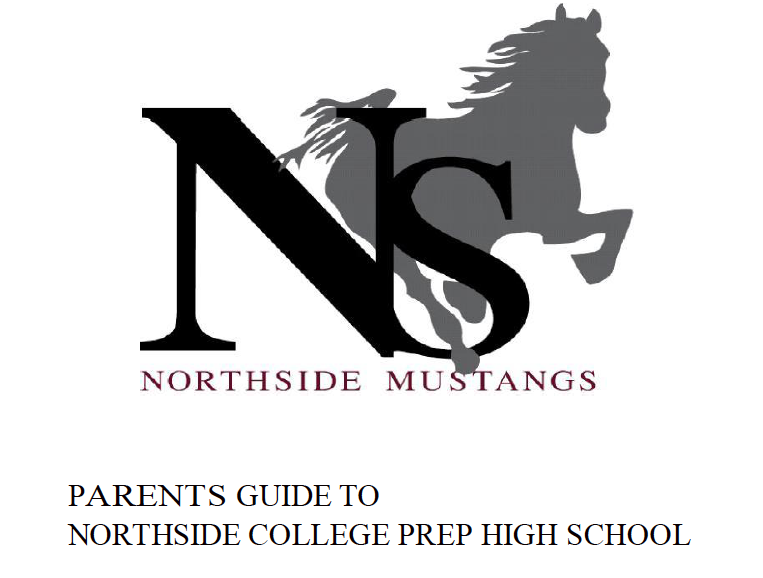# Navigating Northside

Navigating Northside, our parent handbook, is available on the school website under the "Parents" tab. Click HERE to access it directly.

 table div table+table+table+table+table+table+table+table+table+table+table+table+table+table+table+table+table+table+table+table+table+table+table+table+table+table+table+table+table+table+table+table+table+table+table+table+table+table+table+table+table+table+table+table+table+table+table+table+table+table+table+table+table+table+table+table+table+table+table+table+table+table+table+table+table+table+table+table+table div table{width:100%;padding:0}table div table+table+table+table+table+table+table+table+table+table+table+table+table+table+table+table+table+table+table+table+table+table+table+table+table+table+table+table+table+table+table+table+table+table+table+table+table+table+table+table+table+table+table+table+table+table+table+table+table+table+table+table+table+table+table+table+table+table+table+table+table+table+table+table+table+table+table+table+table div table img{width:96.23%;padding:0;float:none}table div table+table+table+table+table+table+table+table+table+table+table+table+table+table+table+table+table+table+table+table+table+table+table+table+table+table+table+table+table+table+table+table+table+table+table+table+table+table+table+table+table+table+table+table+table+table+table+table+table+table+table+table+table+table+table+table+table+table+table+table+table+table+table+table+table+table+table+table+table div table td{width:100%;padding:0 1.88% 18px}/* styles */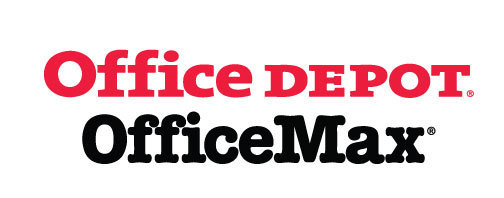# Give Back to Northside

Purchase school and office supplies at Office Depot and OfficeMax and Northside can receive 5% back in credits or FREE supplies. Simply provide our school ID 70031171 at checkout, online, or in stores. There’s no cost to you!

 table div table+table+table+table+table+table+table+table+table+table+table+table+table+table+table+table+table+table+table+table+table+table+table+table+table+table+table+table+table+table+table+table+table+table+table+table+table+table+table+table+table+table+table+table+table+table+table+table+table+table+table+table+table+table+table+table+table+table+table+table+table+table+table+table+table+table+table+table+table+table+table div table{width:100%;padding:0}table div table+table+table+table+table+table+table+table+table+table+table+table+table+table+table+table+table+table+table+table+table+table+table+table+table+table+table+table+table+table+table+table+table+table+table+table+table+table+table+table+table+table+table+table+table+table+table+table+table+table+table+table+table+table+table+table+table+table+table+table+table+table+table+table+table+table+table+table+table+table+table div table img{width:96.23%;padding:0;float:none}table div table+table+table+table+table+table+table+table+table+table+table+table+table+table+table+table+table+table+table+table+table+table+table+table+table+table+table+table+table+table+table+table+table+table+table+table+table+table+table+table+table+table+table+table+table+table+table+table+table+table+table+table+table+table+table+table+table+table+table+table+table+table+table+table+table+table+table+table+table+table+table div table td{width:100%;padding:0 1.88% 18px}/* styles */# Support Northside - Shop AmazonSmile

AmazonSmile is a simple and automatic way to support Northside every time you shop.

Please note that our AmazonSmile name is Friends of "North" "Side" (North Side - two words at AmazonSmile).

 table div table+table+table+table+table+table+table+table+table+table+table+table+table+table+table+table+table+table+table+table+table+table+table+table+table+table+table+table+table+table+table+table+table+table+table+table+table+table+table+table+table+table+table+table+table+table+table+table+table+table+table+table+table+table+table+table+table+table+table+table+table+table+table+table+table+table+table+table+table+table+table+table+table div table{width:100%;padding:0}table div table+table+table+table+table+table+table+table+table+table+table+table+table+table+table+table+table+table+table+table+table+table+table+table+table+table+table+table+table+table+table+table+table+table+table+table+table+table+table+table+table+table+table+table+table+table+table+table+table+table+table+table+table+table+table+table+table+table+table+table+table+table+table+table+table+table+table+table+table+table+table+table+table div table img{width:96.23%;padding:0;float:none}table div table+table+table+table+table+table+table+table+table+table+table+table+table+table+table+table+table+table+table+table+table+table+table+table+table+table+table+table+table+table+table+table+table+table+table+table+table+table+table+table+table+table+table+table+table+table+table+table+table+table+table+table+table+table+table+table+table+table+table+table+table+table+table+table+table+table+table+table+table+table+table+table+table div table td{width:100%;padding:0 1.88% 18px}/* styles */# Follow Us on Social Media

▪ Northside Prep Parents Facebook Page, a closed group for parents, by parents, to share their knowledge of Northside
▪ Friends of Northside Facebook Page, for news everyone can view
 ▪ Northside Prep Parents Facebook Page, a closed group for parents, by parents, to share their knowledge of Northside
 ▪ Friends of Northside Facebook Page, for news everyone can view
 table div table+table+table+table+table+table+table+table+table+table+table+table+table+table+table+table+table+table+table+table+table+table+table+table+table+table+table+table+table+table+table+table+table+table+table+table+table+table+table+table+table+table+table+table+table+table+table+table+table+table+table+table+table+table+table+table+table+table+table+table+table+table+table+table+table+table+table+table+table+table+table+table+table+table+table div table{width:100%;padding:0}table div table+table+table+table+table+table+table+table+table+table+table+table+table+table+table+table+table+table+table+table+table+table+table+table+table+table+table+table+table+table+table+table+table+table+table+table+table+table+table+table+table+table+table+table+table+table+table+table+table+table+table+table+table+table+table+table+table+table+table+table+table+table+table+table+table+table+table+table+table+table+table+table+table+table+table div table img{width:96.23%;padding:0;float:none}table div table+table+table+table+table+table+table+table+table+table+table+table+table+table+table+table+table+table+table+table+table+table+table+table+table+table+table+table+table+table+table+table+table+table+table+table+table+table+table+table+table+table+table+table+table+table+table+table+table+table+table+table+table+table+table+table+table+table+table+table+table+table+table+table+table+table+table+table+table+table+table+table+table+table+table div table td{width:100%;padding:0 1.88% 18px}/* styles */Northside’s website can be found HERE.

Friends of Northside's website can be found HERE.

 table div table+table+table+table+table+table+table+table+table+table+table+table+table+table+table+table+table+table+table+table+table+table+table+table+table+table+table+table+table+table+table+table+table+table+table+table+table+table+table+table+table+table+table+table+table+table+table+table+table+table+table+table+table+table+table+table+table+table+table+table+table+table+table+table+table+table+table+table+table+table+table+table+table+table+table+table+table div table{width:100%;padding:0}table div table+table+table+table+table+table+table+table+table+table+table+table+table+table+table+table+table+table+table+table+table+table+table+table+table+table+table+table+table+table+table+table+table+table+table+table+table+table+table+table+table+table+table+table+table+table+table+table+table+table+table+table+table+table+table+table+table+table+table+table+table+table+table+table+table+table+table+table+table+table+table+table+table+table+table+table+table div table img{width:96.23%;padding:0;float:none}table div table+table+table+table+table+table+table+table+table+table+table+table+table+table+table+table+table+table+table+table+table+table+table+table+table+table+table+table+table+table+table+table+table+table+table+table+table+table+table+table+table+table+table+table+table+table+table+table+table+table+table+table+table+table+table+table+table+table+table+table+table+table+table+table+table+table+table+table+table+table+table+table+table+table+table+table+table div table td{width:100%;padding:0 1.88% 18px}/* styles */